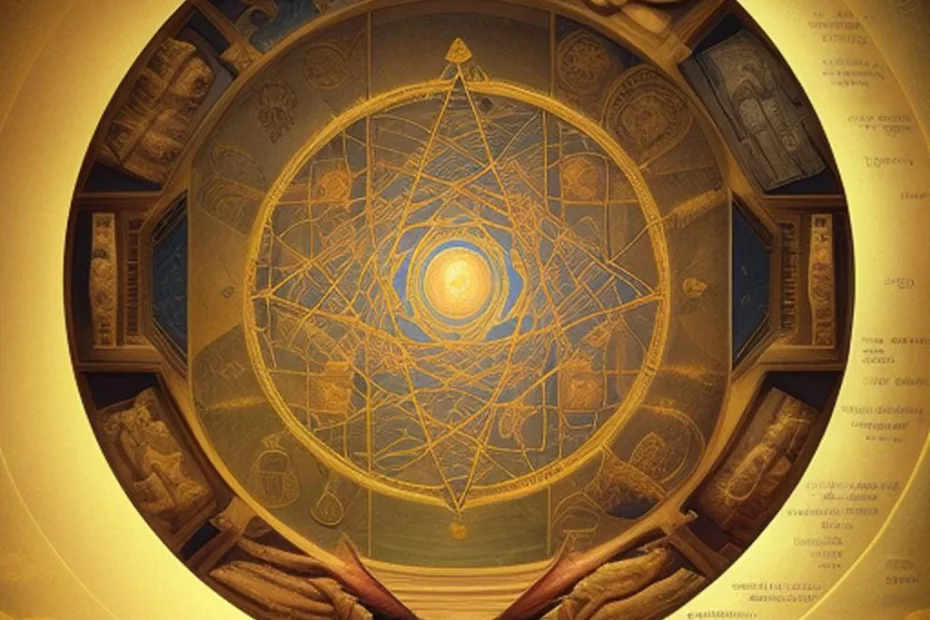# The pursuit of knowledge in mathematics

• Culture, Mathematics## An ancient endeavor that spans across civilizations

The pursuit of knowledge in mathematics is an ancient endeavor that spans across civilizations. The study of mathematics dates back thousands of years, and the insights and discoveries made by ancient mathematicians have laid the foundation for the mathematics we know today.

Many ancient civilizations, such as the Egyptians, Babylonians, Greeks, Indians, and Chinese, contributed to the development of mathematics. They made remarkable discoveries in various branches of mathematics, including arithmetic, geometry, algebra, and trigonometry.

The ancient Egyptians, for example, used mathematics to solve practical problems related to architecture, land surveying, and commerce. They developed techniques for multiplication, division, and solving linear equations. Their knowledge of geometry enabled them to construct pyramids and other impressive structures with great precision.

In ancient Babylon, mathematical tablets have been found containing sophisticated calculations involving numbers and geometric shapes. The Babylonians made significant strides in understanding number systems and worked with fractions and square roots.

The ancient Greeks made enduring contributions to mathematics, producing notable mathematicians such as Pythagoras, Euclid, Archimedes, and Diophantus. Pythagoras is renowned for the Pythagorean theorem, while Euclid’s “Elements” became one of the most influential works in the history of mathematics, establishing the foundations of geometry.

In India, mathematicians like Aryabhata, Brahmagupta, and Bhaskara made significant advances in algebra, trigonometry, and calculus. They developed methods for solving indeterminate equations and studied the properties of triangles and circles.

The Chinese also made significant contributions to mathematics, including developments in arithmetic, algebra, and geometry. They were among the first to explore the use of negative numbers and decimals.

The knowledge and insights of these ancient mathematicians have been passed down through generations, forming the basis of modern mathematics. Today, we continue to build upon the work of these early scholars, exploring new frontiers in mathematics and pushing the boundaries of human understanding.

The continuity of mathematical knowledge from antiquity to the present is a testament to the timeless nature of the pursuit of knowledge. Learning from the wisdom of the ancients allows us to appreciate the rich heritage of mathematics and serves as an inspiration for future generations to continue the legacy of exploration and discovery.The pursuit of knowledge in mathematics. An ancient endeavor that spans across civilizations.

## Geometry one of the foundational branches of mathematics

For ancient civilizations, particularly the Egyptians and the Greeks, geometry was one of the foundational branches of mathematics, and it often preceded the development of other mathematical concepts.

In the ancient world, geometry was intimately connected to practical needs, such as land surveying, architecture, and construction. The Egyptians, for instance, used geometry to design and construct monumental structures like pyramids and temples with incredible precision. The mastery of geometric principles allowed them to create symmetrical and stable buildings that have stood the test of time.

Similarly, the ancient Greeks viewed geometry as a fundamental science and approached it with a philosophical mindset. Geometry played a central role in Greek mathematics, led by mathematicians like Euclid, who formalized the axiomatic approach to geometry in his work “Elements.” Euclid’s “Elements” is a collection of mathematical theorems and proofs that became a seminal work, influencing the development of mathematics for centuries to come.

The Greeks saw geometry as a way to understand the fundamental principles of the universe and to explore the relationships between shapes and forms. This exploration laid the groundwork for their deep investigations into other branches of mathematics, including arithmetic and number theory.

It was only later, in the hands of mathematicians like Pythagoras and his school, that geometry and number theory began to merge, leading to the Pythagorean theorem and the recognition of the mathematical relationship between numbers and geometric figures.

Throughout history, the study of geometry has remained a vital aspect of mathematics education. Even today, geometry plays a crucial role in understanding spatial relationships, symmetry, and various mathematical structures. The geometric insights gained by ancient civilizations have endured, forming the basis for the rich and diverse field of mathematics that continues to evolve and expand.

So, indeed, for the ancient mathematicians, geometry held a foundational place in the development of mathematical knowledge, paving the way for the exploration of other mathematical concepts and setting the stage for the mathematical achievements of subsequent civilizations and eras.

## Shop tips

Knowledge in mathematics on Amazon

Geometry on Amazon

## Thank you for questions, shares and comments! 👍